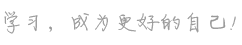• 单元  -1 试听单元
• 物质的变化及性质
• 单元  0 暑假班
• 物质的变化及性质
• 常见的实验操作(一)
• 常见的实验操作(二)
• 空气的组成与氧气含量的测定
• 氧气的性质
• 氧气的实验室制备
• 构成物质的微粒及其性质
• 原子的结构
• 元素和元素元素周期表
• 离子的构成
• 水及水的净化
• 水的组成
• 物质的分类
• 化合价
• 化学式
• 相对分子质量的计算
• 质量守恒定律
• 化学方程式的正确书写
• 化学方程式简单计算
• 碳的单质
• 一氧化碳的性质
• 二氧化碳的性质
• 二氧化碳的实验室制备
• 燃料及燃烧
• 单元  1 秋季班
• 化学实验操作梳理
• 空气中氧气含量的测定
• 氧气的性质及制备实验
• 微观世界知识梳理
• 物质的变化及性质
• 原子和离子
• 常见化学符号的规范性应用
• 有关化学式的计算
• 质量守恒定律
• 化学方程式计算常考题型
• 水的净化
• 水的性质和组成
• 碳单质及一氧化碳
• 还原氧化铜实验
• 二氧化碳的性质及制备
• 燃烧及燃料
• 基本概念既基本原理
• 碳氢氧综合
• 期末冲刺(一)
• 期末冲刺(二)
• 单元  2 寒假班
• 金属材料
• 金属的化学性质
• 金属资源的利用和保护
• 溶液的形成
• 溶液的量度
• 溶解度
• 溶质的质量分数
• 常见的酸和碱
• 酸和酸的通性
• 碱和碱的通性
• 酸碱中和反应
• 生活中常见的盐
• 盐的性质
• 化学肥料
• 酸碱盐的性质（一）
• 酸碱盐的性质（二）
• 化学与生活
• 单元  3 中考一轮复习
• 复分解反应
• 物质的分类及微观粒子
• 常见化学用语及其意义
• 有关化学式的计算
• 质量守恒定律重难点梳理
• 有关化学方程式的计算
• 空气和氧气重难点梳理
• 水的性质和组成重难点梳理
• 碳和一氧化碳重难点梳理
• 二氧化碳的性质和制取
• 溶液和溶液度重难点梳理
• 溶质的质量分数计算
• 溶液计算常考题型
• 金属的化学性质重难点梳理
• 金属的化学性质常考题型
• 酸、碱、盐重难点梳理
• 酸碱盐之间的转化
• 酸碱盐的综合应用（一）
• 酸碱盐的综合应用（二）
• 物质的组成与变化
• 空气、氧气、水
• 碳单质及其化合物
• 气体的制备与净化
• 物质的分离、检验与提纯（一）
• 物质的分离、检验与提纯（二）
• 溶液（一）
• 溶液（二）
• 金属、酸碱盐（一）
• 金属、酸碱盐（二）
• 化学式计算常考题型
• 化学方程式计算常考题型
• 表格、标签型的计算
• 图像型的计算
• 选择题压轴
• 填空简答题压轴
• 物质的推断
• 信息给予题
• 综合性实验
• 探究性实验